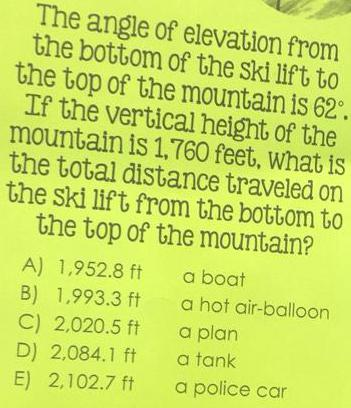Math
Trigonometry
The angle of elevation from the bottom of the ski lift to the top of the mountain is 62°. If the vertical height of the mountain is 1,760 feet, what is the total distance traveled on the ski lift from the bottom to the top of the mountain? A) 1,952.8 ft B) 1,993.3 ft C) 2,020.5 ft D) 2,084.1 ft E) 2,102.7 ft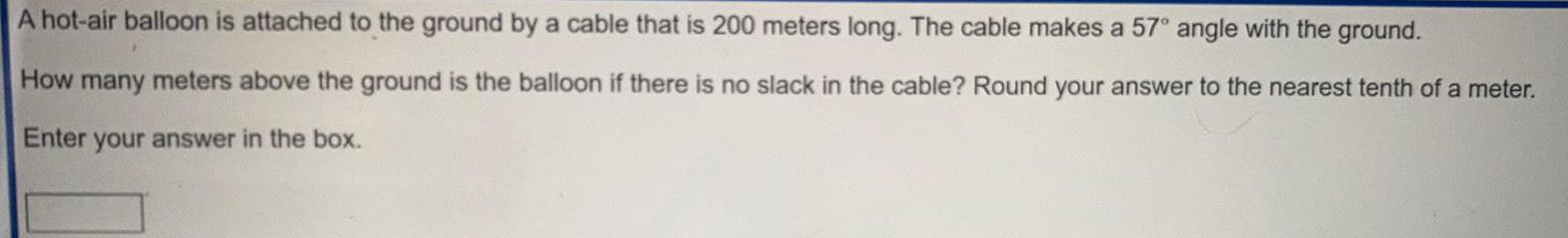Math
Trigonometry
A hot-air balloon is attached to the ground by a cable that is 200 meters long. The cable makes a 57° angle with the ground. How many meters above the ground is the balloon if there is no slack in the cable? Round your answer to the nearest tenth of a meter. Enter your answer in the box.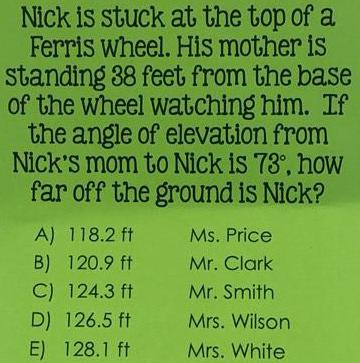Math
Trigonometry
Nick is stuck at the top of a Ferris wheel. His mother is standing 38 feet from the base of the wheel watching him. If the angle of elevation from Nick's mom to Nick is 73°, how far off the ground is Nick? A) 118.2 ft B) 120.9 ft C) 124.3 ft D) 126.5 ft E) 128.1 ft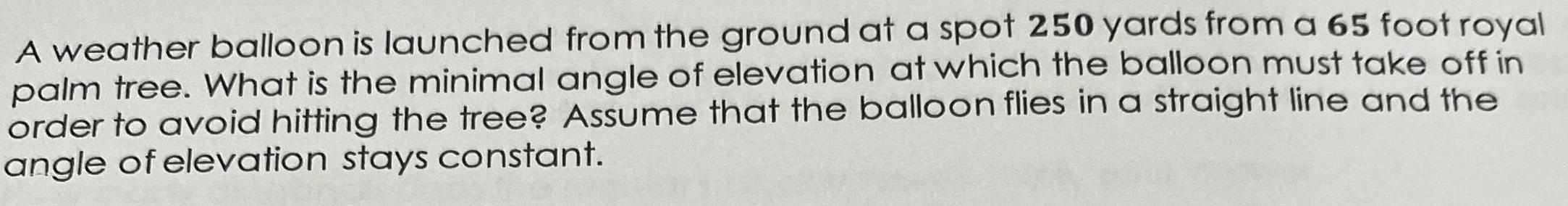Math
Trigonometry
A weather balloon is launched from the ground at a spot 250 yards from a 65 foot royal palm tree. What is the minimal angle of elevation at which the balloon must take off in order to avoid hitting the tree? Assume that the balloon flies in a straight line and the angle of elevation stays constant.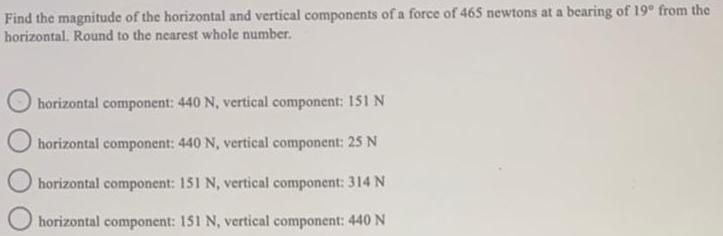Math
Trigonometry
Find the magnitude of the horizontal and vertical components of a force of 465 newtons at a bearing of 19° from the horizontal. Round to the nearest whole number. horizontal component: 440 N, vertical component: 151 N horizontal component: 440 N, vertical component: 25 N horizontal component: 151 N, vertical component: 314 N horizontal component: 151 N, vertical component: 440 N